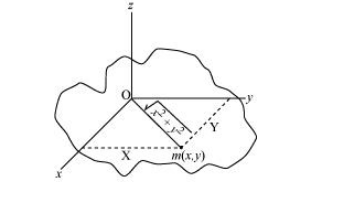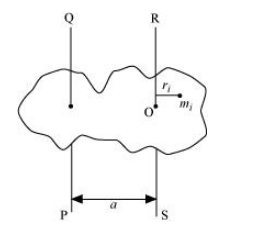# (a) Prove the theorem of perpendicular axes.

Question:

(a) Prove the theorem of perpendicular axes.

(Hint: Square of the distance of a point $(x, y)$ in the $x-y$ plane from an axis through the origin perpendicular to the plane is $\left.x^{2}+y^{2}\right)$.

(b) Prove the theorem of parallel axes.

(Hint: If the centre of mass is chosen to be the origin $\sum m_{i} \mathbf{r}_{i}=0$ ).

Solution:

(a)The theorem of perpendicular axes states that the moment of inertia of a planar body (lamina) about an axis perpendicular to its plane is equal to the sum of its moments of inertia about two perpendicular axes concurrent with perpendicular axis and lying in the plane of the body.

A physical body with centre O and a point mass m,in the xy plane at (xy) is shown in the following figure.Moment of inertia about $x$-axis, $I_{x}=m x^{2}$

Moment of inertia about $y$-axis, $I_{y}=m y^{2}$

Moment of inertia about z-axis, $I_{z}=m\left(\sqrt{x^{2}+y^{2}}\right)^{2}$

$l_{x}+l_{y}=m x^{2}+m y^{2}$

$=m\left(x^{2}+y^{2}\right)$

$=m\left(\sqrt{x^{2}+y^{2}}\right)^{2}$

$I_{\mathrm{x}}+I_{\mathrm{y}}=I_{\mathrm{z}}$

Hence, the theorem is proved.

(b)The theorem of parallel axes states that the moment of inertia of a body about any axis is equal to the sum of the moment of inertia of the body about a parallel axis passing through its centre of mass and the product of its mass and the square of the distance between the two parallel axes.Suppose a rigid body is made up of $n$ particles, having masses $m_{1}, m_{2}, m_{3}, \ldots, m_{n}$, at perpendicular distances $r_{1}, r_{2}, r_{3}, \ldots, r_{n}$ respectively from the centre of mass $\mathrm{O}$ of the rigid body.

The moment of inertia about axis RS passing through the point $\mathrm{O}$ :

$I_{\mathrm{RS}}=\sum_{i=1}^{n} m_{i} r_{i}^{2}$

The perpendicular distance of mass $m_{j}$, from the axis $\mathrm{QP}=a+r_{i}$

Hence, the moment of inertia about axis $Q P$ :

$I_{\mathrm{OP}}=\sum_{i=1}^{n} m_{i}\left(a+r_{i}\right)^{2}$

$=\sum_{i=1}^{n} m_{i}\left(a^{2}+r_{i}^{2}+2 a r_{i}\right)^{2}$

$=\sum_{i=1}^{n} m_{i} a^{2}+\sum_{i=1}^{n} m_{i} r_{i}^{2}+\sum_{i=1}^{n} m_{i} 2 a r_{i}$

$=I_{\mathrm{RS}}+\sum_{i=1}^{\infty} m_{i} a^{2}+2 \sum_{i=1} m_{i} a r_{i}^{2}$

Now, at the centre of mass, the moment of inertia of all the particles about the axis passing through the centre of mass is zero, that is,

$2 \sum_{i=1}^{n} m_{i} a r_{i}=0$

$\because a \neq 0$

$\therefore \sum m_{i} r_{i}=0$

Also,

$\sum_{i=1}^{n} m_{i}=M ; M=$ Total mass of the rigid body

$\therefore I_{\mathrm{OP}}=I_{\mathrm{RS}}+\mathrm{Ma}^{2}$

Hence, the theorem is proved.